# Equal ripple transformer example

Equal-ripple multi-section quarter-wave transformers use the magic of Chebyshev polynomials to find the best approximation of an ideal transformer, subject to the constraint of how many sections you are willing to use. The person that first moved RF engineering toward mathematical synthesis is Wilhelm Cauer, you can meet him in our Microwave Hall of Fame.

We decided to look at transforming 50 ohms to 200 ohms, covering 2-18 GHz. That bandwidth is well known in the defense industry as it covers just about any enemy radar you might encounter in the previous century.  Who knows what new cats are out of the bag once the Taliban starts selling leftover US hardware to China, but electronic warfare is always about upping the game.

Why 200 to 50 ohms?  Because it will make a nice transformer for a four-way power divider.  Think about four, fifty-ohm power amplifiers in parallel... they would present 12.5 ohms to a combined port (50/4). But if you transform them to 200 ohms, they will nicely combine to 50 ohms.  We'll show you this trick on a follow-up page. And don't worry, you won't actually have to realize any impedances higher that 70 or 80 ohms.

We looked at 2, 3, 4, 5, 6, 7 and 8 sections, to see how well the broadband match can be versus how long the impedance transformer is. Note that the center frequency is 10 GHz, so that is where the quarterwave lengths are specified. Everyone will want a good match, but in the end you will pay for it with loss: the longer the transformer, the more loss. Note that the spreadsheet does not include loss.  Just use it to calculate the desired inpedances and then analyze the network further in EDA software such as Microwave Office.

Below is the two-section version. Peak VSWR is 3:22:1. The line impedances are:

Z1=94.137 ohms

Z2=106.228 ohms

Obviously, we would round off the impedances in a real design, but at the synthesis stage there is no reason to.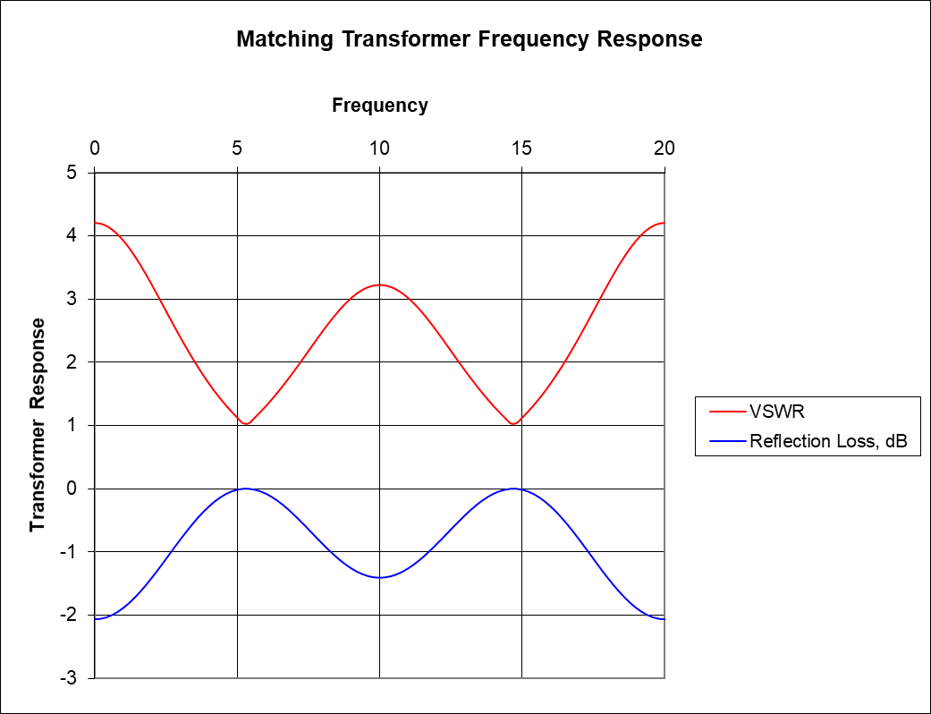Below is the three-section version.  Peak VSWR is 2.63:1. The line impedances are:

Z1=85.697 ohms

Z2=100 ohms

Z3=116.690

What a surprise the middle section of a transformer with an odd number of sections is SQRT(Zin/Zout)... just like in a single section transformer (which also has an odd number of sections).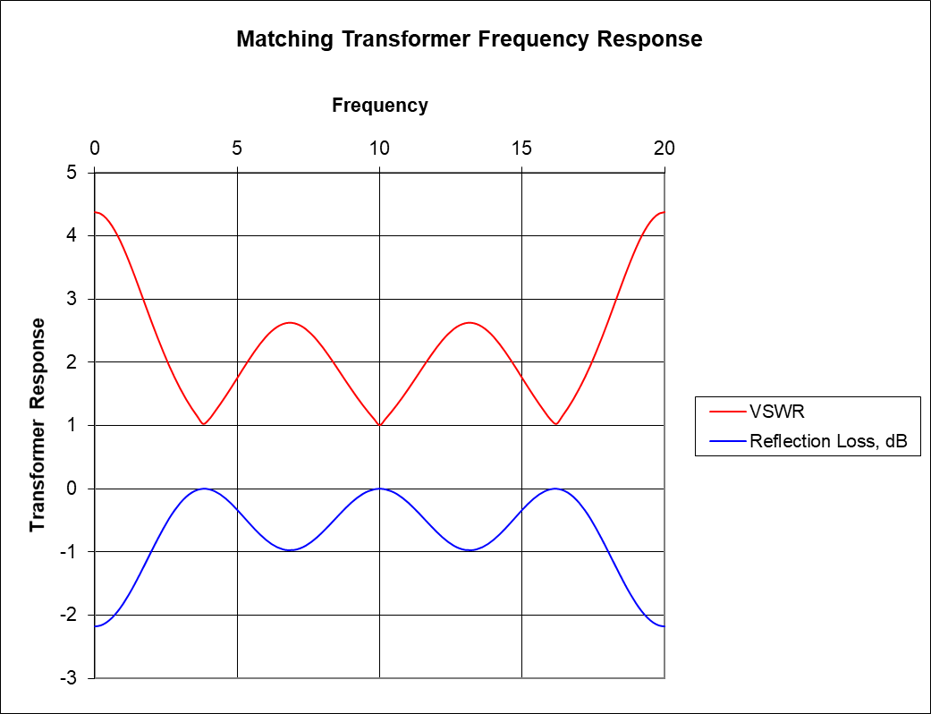Below is the four-section version. Peak VSWR is 2.13:1. The line impedances are:

Z1=77.490 ohms

Z2=91.606 ohms

Z3=109.163 ohms

Z4=129.049 ohms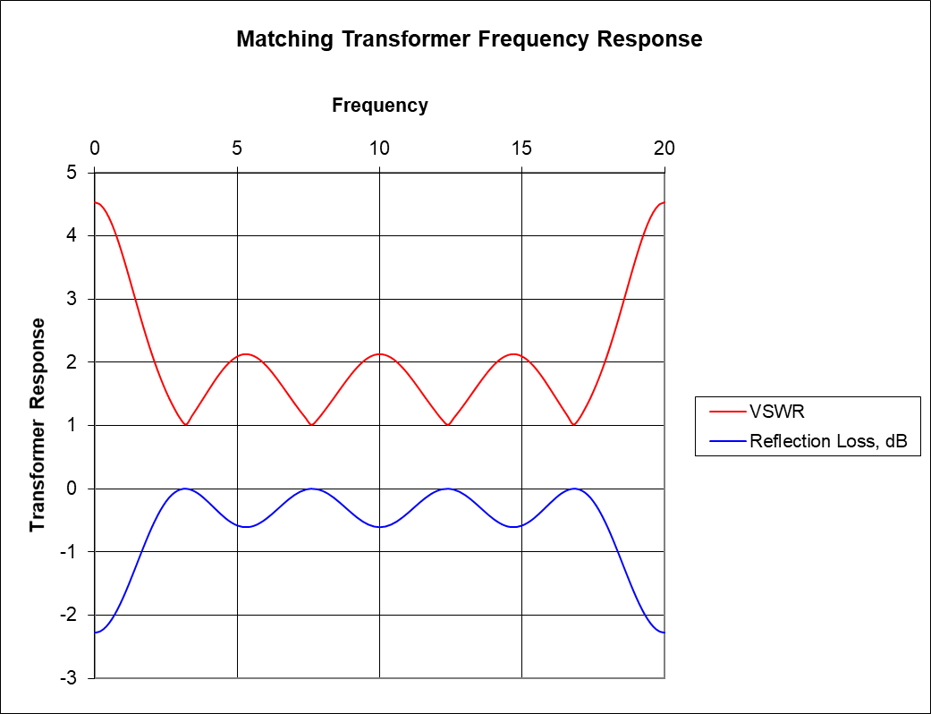Below is the five-section version.  Peak VSWR is 1.78:1. The line impedances are:

Z1=70.704 ohms

Z2=83.424 ohms

Z3=100.000 ohms

Z4=119.869 ohms

Z5=141.434 ohms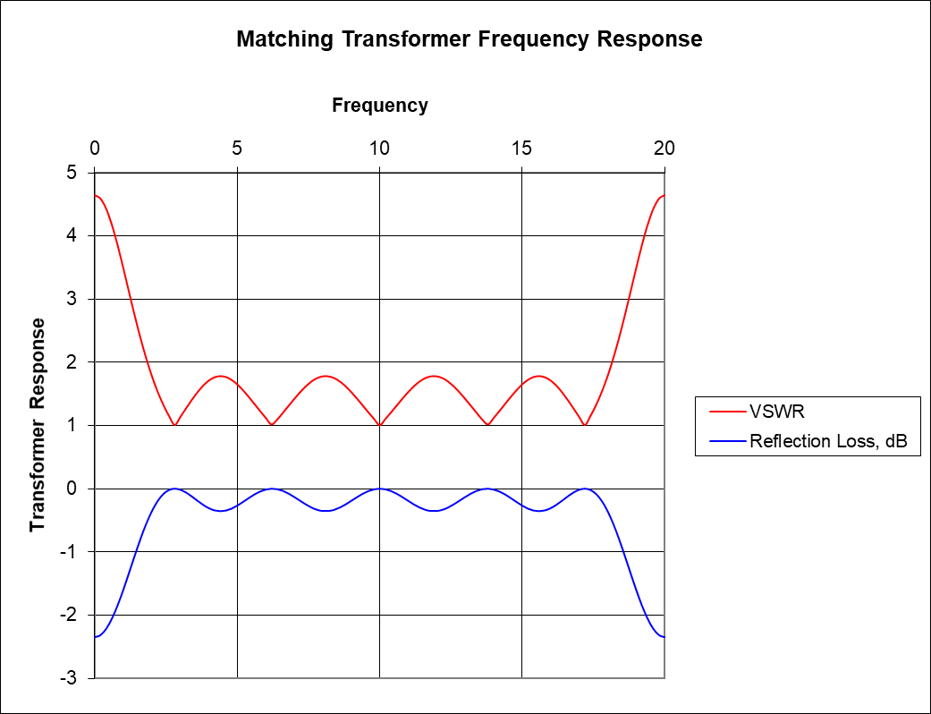Below is the six-section version.  Peak VSWR is 1.54:1. The line impedances are:

Z1=65.479 ohms

Z2=76.421 ohms

Z3=91.187 ohms

Z4=109.664 ohms

Z5=130.854 ohms

Z6=152.721 ohms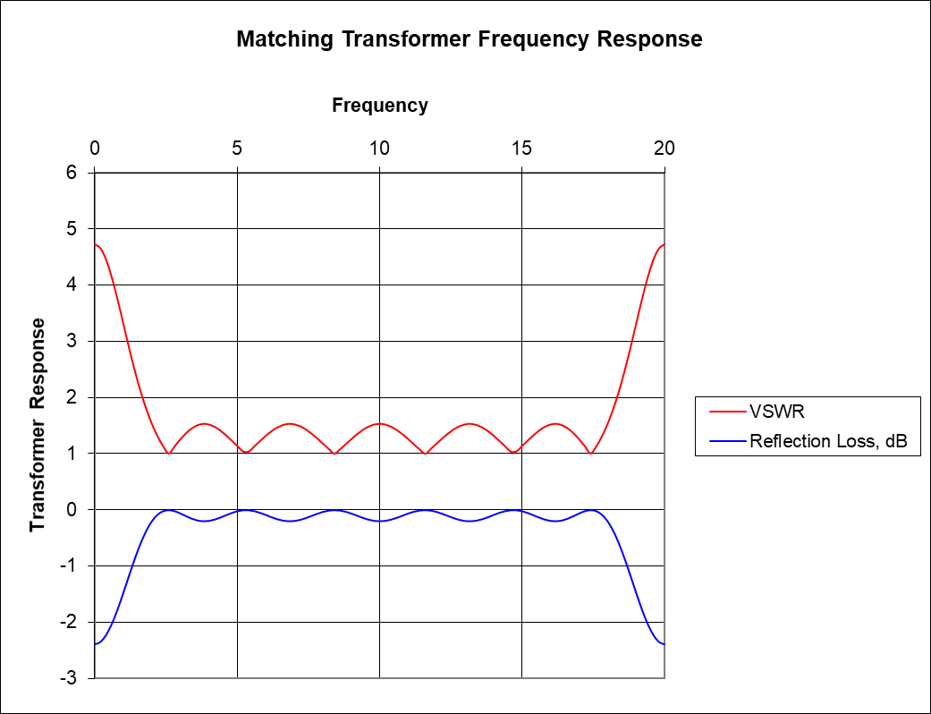Below is the seven-section version.  Peak VSWR is 1.37:1. The line impedances are:

Z1=61.568 ohms

Z2=70.757 ohms

Z3=83.507 ohms

Z4=100.000 ohms

Z5=119.750 ohms

Z6=141.328 ohms

Z7=162.422 ohms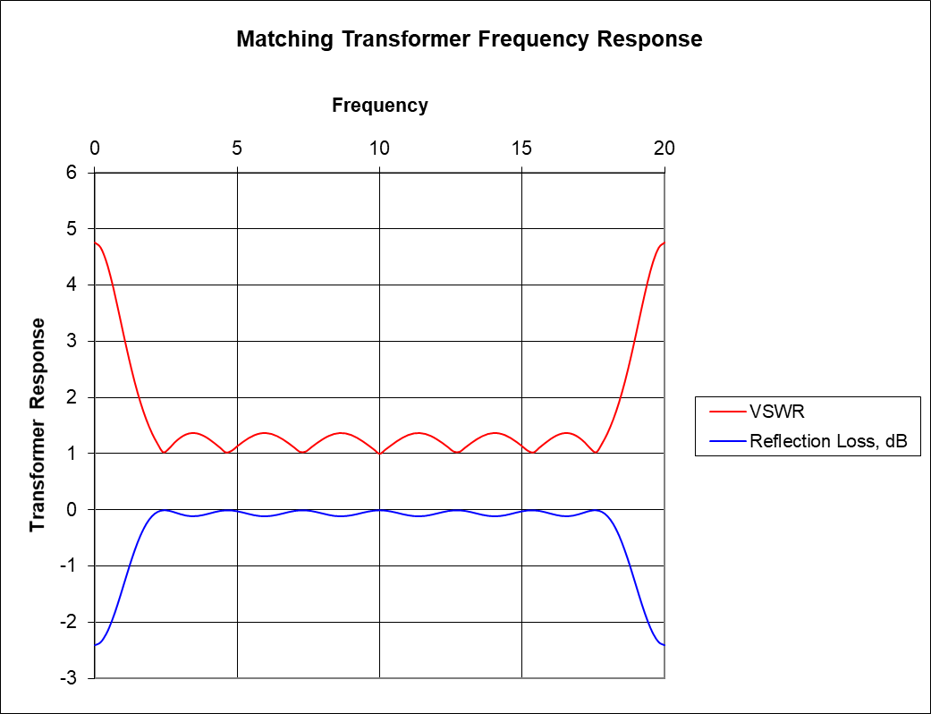Below is the eight section version. Peak VSWR is 1.26:1. The line impedances are:

Z1=58.666 ohms

Z2=66.285 ohms

Z3=77.109 ohms

Z4=91.487 ohms

Z5=109.305 ohms

Z6=129.686 ohms

Z7=150.863 ohms

Z8=170.456 ohms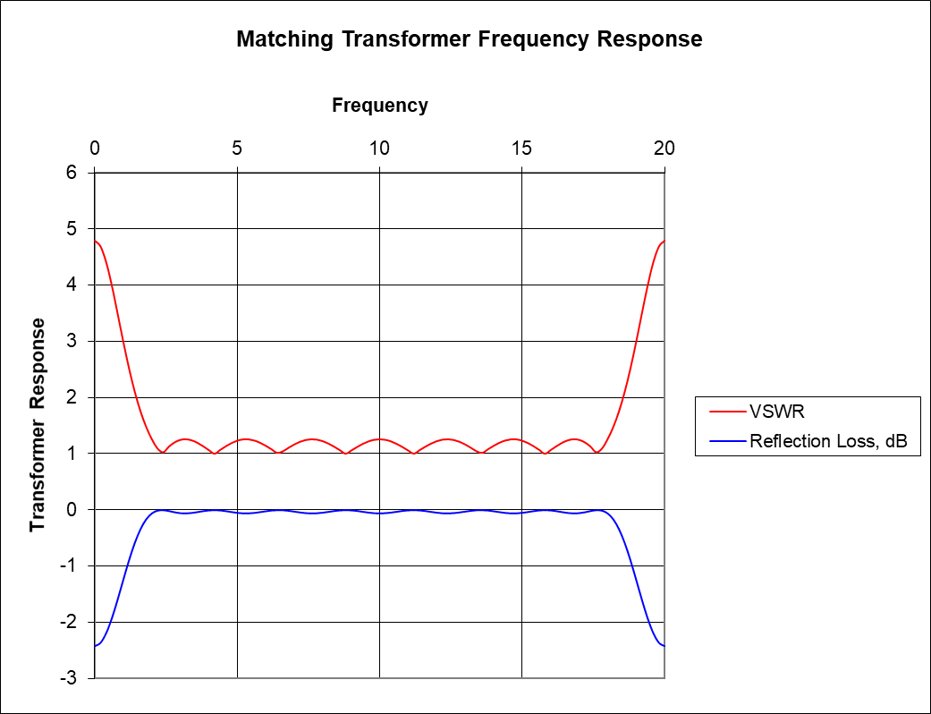Just as a check, we built up the eight-section transformer in Microwave Office. we used the same precision of three decimal digits, even though it is hard to see.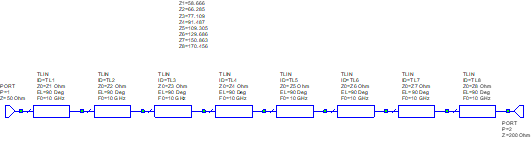Here is Microwave Office prediction, showing the Excel spreadsheet is just about right on the money. We won't worry about the small differences, they might be due rounding errors.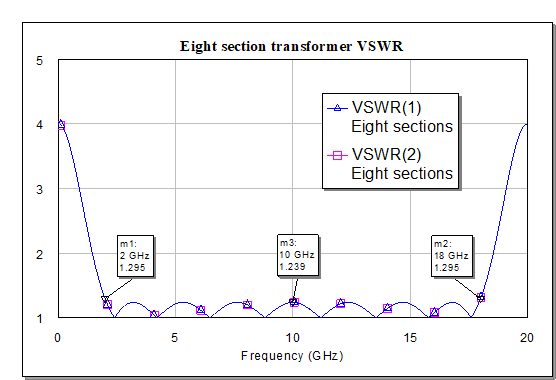At this point, a designer should insert the approximate attenuation of the transmission lines to see the tradeoff between VSWR and insertion loss.  We'll leave that for another day.

Author : Unknown Editor